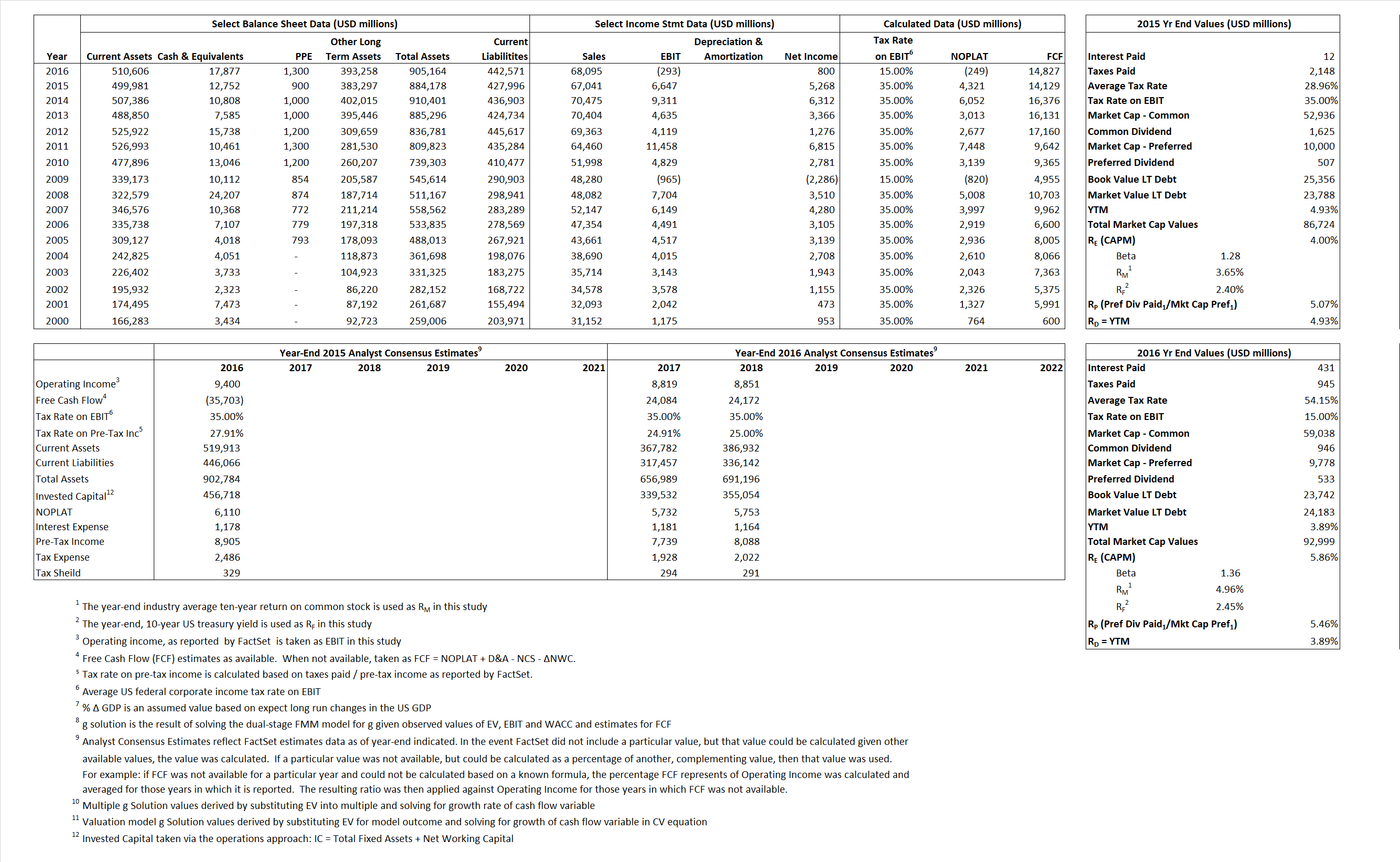# METLIFE

## Analyst Listing

The following analysts provide coverage for the subject firm as of May 2016:

 Broker Analyst Analyst Email Morningstar Brett Horn brett.horn@morningstar.com RBC Capital Markets Eric N. Berg eric.berg@rbccm.com Dowling & Partners Humphrey A. Lee humphrey@dowling.com Argus Research Jacob Kilstein jkilstein@argusresearch.com Sandler O’Neill & Partners John Barnidge jbarnidge@sandleroneill.com Atlantic Equities John Heagerty j.heagerty@atlantic-equities.com Piper Jaffray John Nadel john.m.nadel@pjc.com Daiwa Securities Co. Ltd. Kazuki Watanabe kazuki.watanabe@daiwa.co.jp Janney Montgomery Scott Larry Greenberg lgreenberg@janney.com FBR Capital Markets & Co Randy Binner rbinner@fbr.com Keefe Bruyette & Woods Ryan Krueger rkrueger@kbw.com Raymond James Steven D. Schwartz steven.schwartz@raymondjames.com Deutsche Bank Research Yaron Kinar yaron.kinar@db.com

## Primary Input Data## Derived Input Data

### Equational Form

Net Operating Profit Less Adjusted Taxes NOPLAT  4,321  (249)$NOPLAT\, =\, EBIT\, x\, (1 \,-\, Avg \,\,Tax\,\, Rate\,\, on\,\, EBIT)$
Free Cash Flow FCF 14,129 14,827$FCF\,=NOPLAT\,+\,Non-Cash\,Expenses-\Delta NWC\,-\,NCS$
Tax Shield TS 3 233$TS\,=\,Interest\,\,Paid\,\,x\,\, Avg \,\,Tax\,\,Rate\,\, on\,\, Pre-Tax\,\, Income$
Invested Capital IC 456,182 462,593$IC\,=\,Fixed\,\,Operating\,\,Assets\,\,+\,\,Net\,\, Working\,\, Capital$
Return on Invested Capital ROIC 0.95% -0.05%$ROIC\,=\,\frac { NOPLAT }{ IC }$
Net Investment NetInv  (17,316)  6,411$NetInv\,=\,{ {IC}_{1}}-{{IC}_{0}}+Depreciation$
Investment Rate IR -400.78% -2,574.18%$IR\,=\,\frac {NetInv}{NOPLAT}$
Weighted Average Cost of Capital
WACCMarket  3.99%  4.76%$WACC\,=\,\frac { E }{ V } { R }_{ E }\,+\,\frac { P }{ V } { R }_{ P }\,+\,\frac { D }{ V } { R }_{ D }\left( 1- Avg\,\, Tax\,\,Rate\,\,on\,\,Pre-Tax\,\,Income \right)$
WACCBook    8.24%       1.36%
Enterprise value
EVMarket 73,972  75,122$EV\,=\,Market\,\,Cap\,\,Equity\,+\,\,Long\,\,Term\,\,Debt\,-\,Cash$
EVBook    70,415      74,681
Long-Run Growth
g = IR x ROIC
-3.80%      1.39% Long-run growth rates of the income variable are used in the Continuing Value portion of the valuation models.
g = %$\Delta$ GDP    2.50%     2.50%
Margin from Operations M   9.91%  -0.43%$M\,\,=\,\,\frac{EBIT}{SALES}$
Depreciation/Amortization Rate D   0.00%  0.00%$D\,\,=\,\,\frac{D+A}{EBITDA}$

## Valuation Multiple Outcomes

The outcomes presented in this study are the result of original input data, derived data, and synthesized inputs.

### model g solution

12/31/2015 12/31/2016 12/31/2015 12/31/2016 12/31/2015 12/31/2016

EV/SALES$\frac {EV}{Sales} \,= \,\frac{ROIC\, -\, g}{ROIC\,(WACC\,-\,g)}\,(1\,-\,T)\,(M)$

1.10  1.10  0.36%  -0.99%  -O.05%  -1.10%

EV/EBITDA$\frac {EV}{EBITDA} \,= \,\frac{ROIC\, -\, g}{ROIC\,(WACC\,-\,g)}\,(1\,-\,T)\,(1\,-\,D)$

11.13 -256.39 0.36% -0.99% -O.05% -1.10%

EV/NOPLAT$\frac {EV}{NOPLAT} \,= \,\frac{ROIC\, -\, g}{ROIC\,(WACC\,-\,g)}$

17.12 -301.63 0.36% -0.99% -O.05% -1.10%

EV/FCFOPS$\frac {EV}{FCF_{OPS}} \,= \,\frac{ROIC\, -\, g}{ROIC\,(WACC\,-\,g)}\,(1\,-\,T)$

5.24 5.07 0.36% -0.99% -O.05% -1.10%

EV/EBIT$\frac {EV}{EBIT} \,= \,\frac{ROIC\, -\, g}{ROIC\,(WACC\,-\,g)}\,(1\,-\,T)$

11.13 -256.39 0.36% -0.99% -O.05% -1.10%

EV/IC$\frac {EV}{IC} \,= \,\frac{ROIC\, -\, g}{WACC\,-\,g}$

0.16 0.16 0.36% -0.99% -O.05% -1.10%